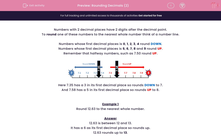# Rounding Decimals (2)

In this worksheet, students must round numbers with two decimal places to the nearest whole number.Key stage:  KS 2

Curriculum topic:   Maths and Numerical Reasoning

Curriculum subtopic:   Decimals

Difficulty level:#### Worksheet Overview

Numbers with 2 decimal places have 2 digits after the decimal point.

To round one of these numbers to the nearest whole number think of a number line.

Numbers whose first decimal places is 01, 2, 3, 4 round DOWN.

Numbers whose first decimal places is 5, 6, 7, 8 and 9 round UP.

Remember that halfway numbers, such as 7.50 round UP.Here 7.35 has a 3 in its first decimal place so rounds DOWN to 7.

And 7.58 has a 5 in its first decimal place so rounds UP to 8.

Example 1

Round 12.63 to the nearest whole number.

12.63 is between 12 and 13.

It has a 6 as its first decimal place so rounds up.

12.63 rounds up to 13.

Example 2

Round 10.38 to the nearest whole number.

10.38 is between 10 and 11.

It has a 3 as its first decimal place so rounds down.

10.38 rounds down to 10.

Example 3

Round 16.50 to the nearest whole number.